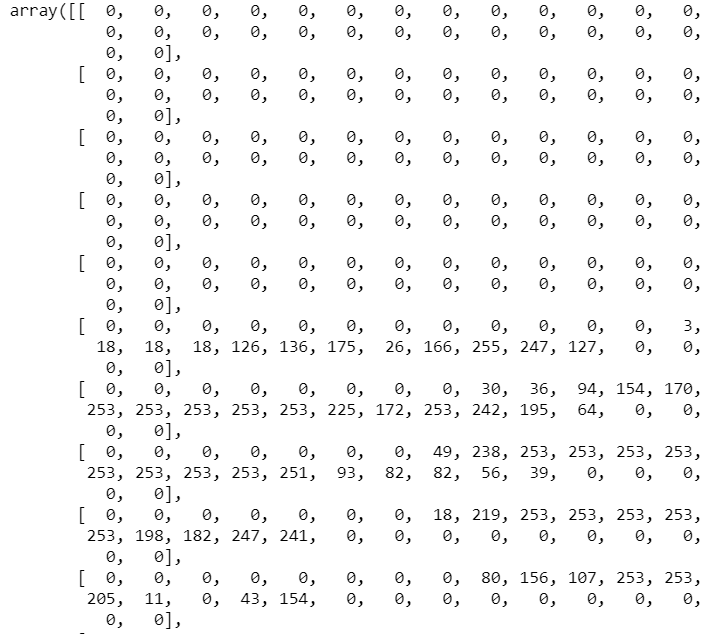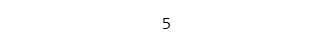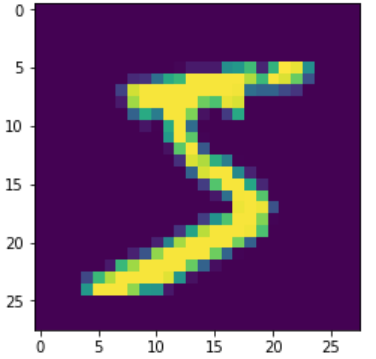# Classify Hand-Written Digits Using Python and Convolutional Neural NetworksShare :# Start Programming:

```# Description: This program uses Convolutional Neural Networks (CNN)
#              to classify handwritten digits as numbers 0 - 9```
`pip install tensorflow keras numpy matplotlib`
```#import the libraries
from keras.models import Sequential
from keras.layers import Dense, Conv2D, Flatten
from keras.datasets import mnist
from keras.utils import to_categorical
import matplotlib.pyplot as plt
import numpy as np```
```#Load the data and split it into train and test sets
```#Get the image shape
print(X_train.shape)
print(X_test.shape)```
`X_train`Sample of the first image in the training data set as an array
```#Print the image label
y_train```The label of the first image in the training data setThe image of the 1st hand written digit in the training set
```#Reshape the data to fit the model
X_train = X_train.reshape(60000, 28,28,1)
X_test = X_test.reshape(10000, 28, 28, 1)```
```#One-Hot Encoding
y_train_one_hot = to_categorical(y_train)
y_test_one_hot = to_categorical(y_test)

#Print the new label
print(y_train_one_hot)```
`model = Sequential()model.add(Conv2D(64, kernel_size=3, activation='relu', input_shape=(28,28,1)))model.add(Conv2D(32, kernel_size=3, activation='relu'))model.add(Flatten())model.add(Dense(10, activation='softmax'))`
`#Compile the model model.compile(optimizer='adam', loss='categorical_crossentropy', metrics=['accuracy'])`
`hist = model.fit(X_train, y_train_one_hot, validation_data=(X_test, y_test_one_hot), epochs=3)`
```#Visualize the models accuracy
plt.plot(hist.history['acc'])
plt.plot(hist.history['val_acc'])
plt.title('Model Accuracy')
plt.ylabel('Accuracy')
plt.xlabel('Epoch')
plt.legend(['Train', 'Val'], loc='upper left')
plt.show()```
```predictions = model.predict(X_test[:4])
predictions```
```#Print our predicitons as number labels for the first 4 images
print( np.argmax(predictions, axis=1))
#Print the actual labels
print(y_test[:4])```
```#Show the first 4 images as pictures
for i in range(0,4):
image = X_test[i]
image = np.array(image, dtype='float')
pixels = image.reshape((28,28))
plt.imshow(pixels, cmap='gray')
plt.show()```